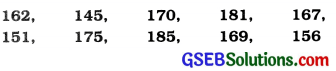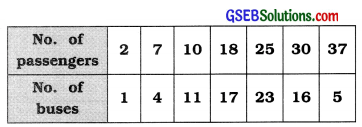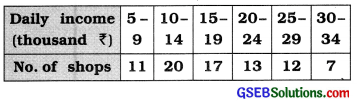# GSEB Solutions Class 11 Statistics Chapter 4 Measures of Dispersion Ex 4.1

Gujarat Board Statistics Class 11 GSEB Solutions Chapter 4 Measures of Dispersion Ex 4.1 Textbook Exercise Questions and Answers.

## Gujarat Board Textbook Solutions Class 11 Statistics Chapter 4 Measures of Dispersion Ex 4.1

Question 1.
The following data refer to the heights in cms. of 10 students of a class. Find range and coefficient of range of height of the students.Here, xH = 185 cm; xL=145cm

Range of height:
R = xH – xL
= 185 – 145
= 40 cm
Coefficient of range = $$\frac{x_{\mathrm{H}}-x_{\mathrm{L}}}{x_{\mathrm{H}}+x_{\mathrm{L}}}$$
= $$\frac{185-145}{185+145}$$
= $$\frac{40}{330}$$
= 0.12Question 2.
A bus company has 77 buses for travelling in the city. The information of number of passengers in bus on a particular day at a particular time is given below. Find the range and coefficient of range of number of passengers.Here. XH = Maximum passengers travelling in a bus = 37
XL = Minimum passengers travelling in a bus = 2

Range of number of passengers:
R = xH – xL
= 37 – 2
= 35 Passengers

Coefficient of range = $$\frac{\mathrm{R}}{x_{\mathrm{H}}+x_{\mathrm{L}}}$$
= $$\frac{35}{37+2}$$
= $$\frac{35}{39}$$
= 0.90

Question 3.
Using the following frequency distribution of marks of students of a school, find range and relative range of the marks:Here, xH = Upper limit of the last class 70 – 80 = 80 marks
xL = Lower limit of the initial class 20 – 30 = 20 marks

Range of marks of students :
R = xH – xL
= 80 – 20 = 60 marks

Relative range of marks:
Relative range = $$\frac{\mathrm{R}}{x_{\mathrm{H}}+x_{\mathrm{L}}}$$
= $$\frac{60}{80+20}$$
= $$\frac{60}{100}$$
= 0.60Question 4.
The frequency distribution of daily income , (in thousand of 80 shops of an area is as follows. Find the absolute and the relative measure of range of daily income from it.Coefficient of range = $$\frac{x_{\mathrm{H}}-x_{\mathrm{L}}}{x_{\mathrm{H}}+x_{\mathrm{L}}}=\frac{\mathrm{R}}{x_{\mathrm{H}}+x_{\mathrm{L}}}$$
= $$\frac{29}{34+5}$$
= $$\frac{29}{39}$$